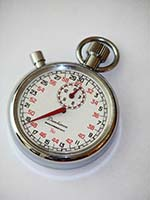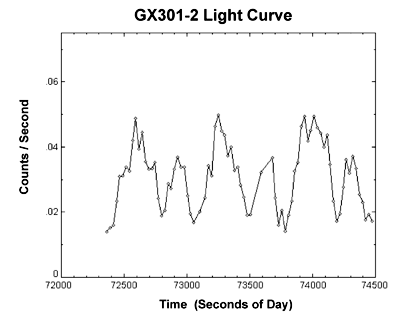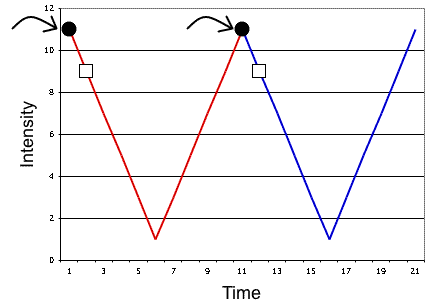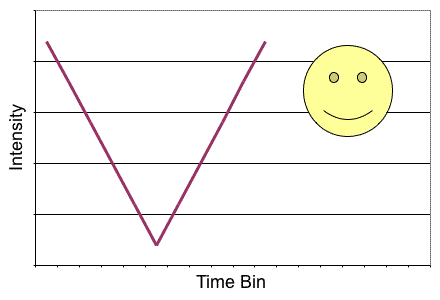# National Aeronautics and Space Administration

## Goddard Space Flight Center## Lesson Plans: Time that Star! (Day 2)# Time That Star!

## Day 2

### Summary

On Day 2 of this lesson, students look at data collected by NASA satellites to find periodic motion in the sources. Using their knowledege of pendulums and periodic motion learned on Day 1 and a technique knows as "folding", they charaterize the period of astronomical sources.

### Materials

• Teacher
• Texas Instruments 83 graphing calculator
• Optional Introdution Demonstration
• Calculator Based Laboratory with light probe
• CHEMBIO program
• Binary Star Data Set (html, Word, PDF)
• Binary Star Plot Set (html, Word, PDF)
• Sample of Epoch Folding (html, Word, PDF)
• For each triad of students
• Texas Instruments 83 graphing calculator
• Calculator
• Metric ruler
• Graph paper
• Red and blue pencils
• Handouts:

### Introduction

Students should again be encouraged to discuss the definition of a cycle or periodicity. Students should recall the lab that was completed on Day 1 and how it relates to determining the "period of the periodicity". In the lab, they should have determined the length of time it took the pendulum to complete one full cycle of its movement. That length of time is called the "period" of the pendulum's behavior.

The students should now be ready to take all of the information they learned on Day 1 and apply it to data received by high-energy satellites from various binary star systems. By properly analyzing the data, they will be able to determine the rotation period of a star and/or the binary orbital period of the stellar system.

The astronomical objects used in this lesson may be unfamiliar to you and your students.You can read all about these objects at:

### Optional engagment using the CHEMBIO program on a TI-83 graphing caluculator

An excellent way for students to visualize the behavior of a pulsar and its relation to a light curve is by using a student holding a flashlight, the Calculator Based Laboratory (CBL), its light probe, the CHEMBIO program, and the TI-83 graphing calculator. Have the student hold the light and rotate around with it pointed out toward his classmates, sweeping across the light probe as s/he rotates. As the rotating beam of light sweeps past the observer or the probe, they are able to detect an increase in the intensity of light they see from the flashlight. By observing this brightness over time and plotting it, a periodic behavior will be seen. The period of the behavior is a measure of the spin rate of the person holding the flashlight. Have you students then consider how this demonstration provides insight into the behavior of pulsars.

Tell me how to set up and run the CHEMBIO program

For the next part of the lesson, students will look closely at astronomical data to determine the period and apply some mathematical methods in the process.

### Guided Practice

Ask students, "What would we do with data like these?"Light curve of GX 301-2's stellar rotation retrieved from the HEAO-1 satellite. GX 301-2 is a system where a rotating neutron star is in orbit with a supergiant B star.

Ask, "Do you see the measured brightness of the source 'repeating' itself? Where? Describe what seems to be happening", and "Can you determine the period here?".

By being able to interpret the graphed data, students will hopefully respond with answers close to 700 seconds – which is the correct length of the period. But now tell students that there has to be a way to be more mathematical method to determining a period within a set of data – something better than just guessing somthing close!

### Procedure

Here are some sample data points for intensity (in increments of 100,000) over time (in months). Is there periodic behavior in these data? If so, what is the period?

 Time 1 2 3 4 5 6 7 8 9 10 11 12 Intensity 11 9 7 5 3 1 3 5 7 9 11 9 Time 13 14 15 16 17 18 19 20 21 22 23 24 Intensity 7 5 3 1 3 5 7 9 11 9 7 5

Students should copy these data on their paper and create a line plot on graph paper.

Now the students are ready to determine the true, mathematical period through the method called Epoch Folding. The following 8 steps are for the student to follow. You may want to make photo copies or instruct students to copy them from the overhead.

1. Guess the length of the period.
2. Locate the section of the plot for your guess of a period, starting with the smallest time on the x-axis. Color over the line it makes with a red pencil.
3. Locate the next period segment which should be an exact and adjacent match to the curve of the first period. Color over the line the second period makes with a blue pencil.
4.Graph of the sample data with the first period shown in red and the second period shown in blue.

5. Starting from the left end of the x-axis, find the 1st data point on the red curve and the 1st data point on the blue curve and determine the mean of their y values (in this case, intensity is the y value). For this first data point, we will call its x-axis value "Time Bin #1".

Continue to do this with the remaining points on the curves, calling the x-axis values "Time Bin #2", "Time Bin #3", etc. and associating the y value of mean with the x value "Time Bin".

6.The two black dots represent the first locations on adjacent curves. The white squares represent the second two.

7. Create a chart that looks like this and import the appropriate, calculated data.
8. Time BinIntensity
1
2
3
4
5
6
7
8
9
etc.
9. Plot the data from that chart.
10. Does it make a curve? Does it resemble the plot of your guessed period? If so, you have the period!
11.The resutling graph if the initial guess for the object's period was a good one will resemble the period of the object you saw in the original data.

12. Does it make a straight line, or an approximate straight line? If so, the period you guessed is wrong and you need to go back to step one of the Epoch Folding instructions and begin again.
13.The resutling graph if the initial guess for the object's period was a bad one will be an approximate straight line and will not resemble the period you saw in your original data.

14. This method can be used with any periodic data set, though most data sets won't be as clean as this sample set!

The correct period for these data is 11 months. Be sure to show students both an example of correct Epoch Folding and an example incorrect Epoch Folding as well.

Tell students that they now need to copy (or have a photocopy of) the GX301-2 data and create a line plot on graph paper.

Next tell students to use Epoch Folding to confirm the period they guessed for GX301-2.

After guessing the length of the period and binning the data, students should arrive at the answer of 700 seconds. That is, the pulsar is rotating and completing its cycle every 700 seconds.

### Guided Practice: Optional Procedure

Another option for this lesson is to follow the procedure of Epoch Folding, but with the teacher using a graphing calculator.

Tell me how to do this.

### Independent Practice

The students are now ready to complete Epoch Folding on the data of each binary star system listed below.

1. Stellar Rotation Period

Have students determine the stellar rotation periods of GX 301-2 and Cen X-3 data using the provided data for each.

GX 301-2 is a system where a rotating neutron star is in orbit with a supergiant B star. The stellar rotational period data were retrieved from the HEAO-1 satellite.

Cen X-3 is a system where a pulsar is in orbit around a 17 solar mass (17x the mass of the Sun) O star (very large blue star). This is a peculiar source where long term variations are seen in both the pulsar period and the orbital period. It has been hypothesized that this is due to a third "body" that may exist within this system. The stellar rotation data were retrieved from the EXOSAT.

2. Orbital Period

Have students determine the orbital periods of Cir X-1 and GX 301-2 using the provided data for each.

Cir X-1 is a system where a neutron star is in orbit with a low mass companion (possibly an M star). The Cir X-1 orbital data were retrieved from the RXTE satellite's All Sky Monitor. The rotational period of the neutron star is currently not known.

GX 301-2 is a system where a rotating neutron star is in orbit with a supergiant B star. The orbital period data were retrieved from the Vela 5B satellite's All Sky Monitor.

The students are ready to determine the orbital and stellar rotation periods through the method of Folding. The 8 steps of Folding should be used. It is probably worthwhile to review these steps at this point.

Instruct the students to use Epoch Folding on the data sets in the following order:

1. The Cen X-3 stellar rotation period data, trying the following periods; start at 3.6 seconds and add multiples of 0.6 seconds after that, such as 3.6, 4.2, 4.8, etc.
2. ### Show me Cen X-3's stellar rotation period

The pulsar rotation period of Cen X-3 is 4.8 seconds.

3. The GX 301-2 stellar rotation period data, trying the following periods; start at 650 seconds and add multiples of 25 seconds after that, such as 650, 675, 700 etc.
4. ### Show me GX 301-2's stellar rotation period

The stellar rotation period of GX 301-2 is 700 seconds

5. The Cir X-1 orbital period data, trying the following periods; start at 14.6 days and add single days after that, such as 14.6, 15.6, 16.6, etc.
6. ### Show me Cir X-1's rotation period

The orbital period if Cir X-1 is 16.6 days.

7. The GX 301-2 orbital period data, trying the following periods; start at 35.5 days and add multiples of 3 days after that, such as 35.5, 38.5, 41.5, etc.
8. ### Show me GX 301-2's orbital period

The orbital period of GX 301-2 is 41.5 days.

### Assessment

Formative assessment and observation should be evident throughout the lesson. In addition, you may want students to fill out the Time That Star! Day 2 Wrap-up worksheet. Finally, bring students together to discuss their results to see if everyone agrees on the rotation and orbital periods they determined.

### Extension: Using Student Hera to Analyze More Data Sets

Student Hera gives students the opportunity to analyze the same data sets that scientists use, using the same tools that scientists use. The Student Hera web pages walk students through examining the data files and finding the periods using epoch folding tools.